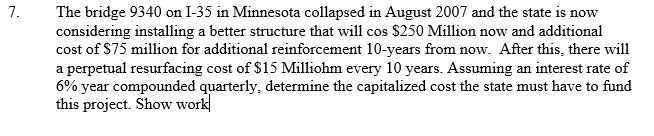Homework Help Question & Answers

# 7. The bridge 9340 on I-35 in Minnesota collapsed in August 2007 and the state is now considering...7. The bridge 9340 on I-35 in Minnesota collapsed in August 2007 and the state is now considering installing a better structure that will cos \$250 Million now and additional cost of S75 million for additional reinforcement 10-years from now. After this, there will a perpetual resurfacing cost of \$15 Milliohm every 10 years. Assuming an interest rate of 6% year compounded quarterly, determine the capitalized cost the state must have to fund this project. Show work

#### Homework Answers

ReportAnswer #1

i = 6% compounded quarterly = 6%/4 = 1.5% per quarter

Effective rate = (1+0.015)^4 - 1 = 0.06136355 = 6.136355%

CC = 250m + 75m *(P/F,6.136355%,10) + 15m *(A/F,6.136355%,10)*(P/F,6.136355%,10) / 0.06136355

(A/F,6.136355%,10) = i/((1 + i)^n-1) = 0.06136355 / (( 1 +0.06136355)^10 -1) = 0.07538349

(P/F,6.136355%,10) = (1 + i)^-n = (1 + 0.06136355)^-10 = 0.55126232

CC = 250000000 + 75000000 * 0.55126232 + 15000000 * [0.07538349*0.55126232 / 0.06136355]

CC = 250000000 + 41344674 + 10158166.60

CC = 301502840.60

CC = 301.50 m

Add Homework Help Answer
##### Add Answer of: 7. The bridge 9340 on I-35 in Minnesota collapsed in August 2007 and the state is now considering...
Your Answer: Your Name: What's your source?
Not the answer you're looking for? Ask your own homework help question. Our experts will answer your question WITHIN MINUTES for Free.
More Homework Help Questions Additional questions in this topic.

• #### A 125-ft diagonal brace on a bridge connects a support of the center of the bridge to a side support on the bridge

Need Online Homework Help?

Get FREE EXPERT Answers
WITHIN MINUTES
Related Questions